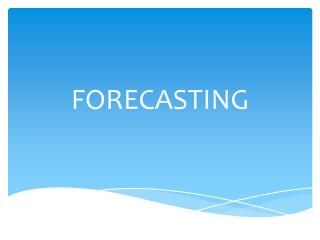DownloadDownload PresentationFORECASTING

# FORECASTING

Télécharger la présentation## FORECASTING

- - - - - - - - - - - - - - - - - - - - - - - - - - - E N D - - - - - - - - - - - - - - - - - - - - - - - - - - -
##### Presentation Transcript

1. FORECASTING

2. Minimum Mean Square Error Forecasting Based on the available history of the series up to time t, namely Y1, Y2,…, Yt− 1, Yt, we would like to forecast the value of Yt+ lthat will occur l time units into the future. We call time t the forecast origin and l the lead time for the forecast, and denote the forecast itself as The minimum mean square error forecast is given by

3. ARIMA Forecasting We shall first illustrate many of the ideas with the simple AR(1) process with a nonzero mean that satisfies Consider the problem of forecasting one time unit into the future. Replacing t by t + 1 in Equation (9.3.1), we have AR(1)

4. Equation (9.3.7), which is recursive in the lead time l, shows how the forecast for any lead time l can be built up from the forecasts for shorter lead times by starting with the initial forecast computed using Equation (9.3.6). The forecast is then obtained from , Then ) from ) , and so on until the desired is found

5. However, Equation (9.3.7) can also be solved to yield an explicit expression for the forecasts in terms of the observed history of the series. Iterating backward on l in Equation (9.3.7), we have

6. As a numerical example, consider the AR(1) model that we have fitted to the industrial color property time series. The maximum likelihood estimation results were partially shown in Exhibit 7.7

7. For illustration purposes, we assume that the estimates φ = 0.5705 and μ = 74.3293 are true values. The final forecasts may then be rounded The last observed value of the color property is 67, so we would forecast one time period ahead as†

8. Alternatively, we can use Equation (9.3.8): For lead time 2, we have from Equation (9.3.7)

9. which is very nearly μ (= 74.3293) At lead 5, we have and by lead 10 the forecast is

10. However, for an invertible model, etis a function of Y1, Y2, …, Ytand so MA(1) consider the MA(1) case with nonzero mean: Again replacing t by t + 1 and taking conditional expectations of both sides, we have

11. Using Equations (9.3.19) and (9.3.20), we have the one-step-ahead forecast for an invertible MA(1) expressed as one-step-ahead forecast error is

12. For longer lead times, we have But, for l > 1, both et + land et + l − 1 are independent of Y1, Y2,…, Yt. Consequently,theseconditional expected values are the unconditional expected values, namely zero, and we have

13. ARMA(1,1) model. We have With and, more generally, using Equation (9.3.30) to get the recursion started.

14. Equations (9.3.30) and (9.3.31) can be rewritten in terms of the process mean and then solved by iteration to get the alternative explicit expression

15. ARIMA(1,1,1) so that Thus

16. Prediction Limits For a given confidence level 1 − α, we could use a standard normal percentile, z1 − α/2, to claim that or, equivalently,

17. Thus we may be (1 − α)100% confident that the future observation Yt+ lwill be contained within the prediction limits

18. Forecasting Transformed Series Differencing Suppose we are interested in forecasting a series whose model involves a first difference to achieve stationarity. Two methods of forecasting can be considered: forecasting the original nonstationary series, for example by using the difference equation form of Equation (9.3.28), with φ’s replaced by ϕ’s throughout, or forecasting the stationary differenced series Wt= Yt− Yt− 1 and then “undoing” the difference by summing to obtain the forecast in original terms.

19. Log Transformations As we saw earlier, it is frequently appropriate to model the logarithms of the original series—a nonlinear transformation. Let Ytdenote the original series value and let Zt=log(Yt). It can be shown that we always have

20. Thus, the naive forecast exp[ (l)] is not the minimum mean square error forecast of Yt+ l. To evaluate the minimum mean square error forecast in original terms, we shall find the following fact useful: If X has a normal distribution with mean μ and variance , then